# Continuous analogues of iteration methods

Continuous models that make it possible to study problems concerning the existence of solutions of non-linear equations, to produce by means of the well-developed apparatus of continuous analysis preliminary results on the convergence and optimality of iteration methods, and to obtain new classes of such methods.

One can set up a correspondence between methods for solving stationary problems by adjustment (see Adjustment method) and certain iteration methods (see , ). For example, for the solution of a linear equation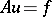(1)

with a positive-definite self-adjoint operatorit is known that one-step iteration methods of the form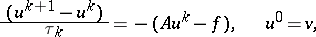(2)

converge for sufficiently small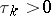. Introduce a continuous timeand regard the quantities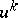as the values of a certain function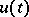at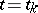, where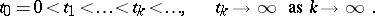If one puts, whereis a continuous function for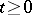, then in passing to the limit in (2) as, one obtains a continuous analogue of the iteration method (2):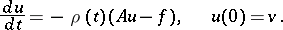(3)

If alsoas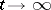, thentends to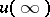, a solution of (1).

Similarly, with the one-step gradient iteration methods for the minimization of a function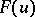: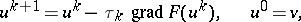(4)

one can associate a continuous analogue:(5)

Here the functionaffects only the parametrization of the curve of steepest descent. To solve (1) one may take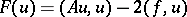. Then the formulas (4) take the form (2) and the equations (5) the form (3).

By means of transformations two-step iteration methods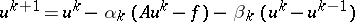(6)

can be brought to the form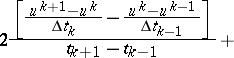(7)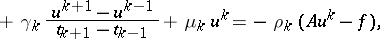where the quantities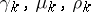, andare (non-uniquely) defined in terms of the parametersandof (6). Taking limits in (7) as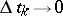leads to a continuous analogue:(8)

The adjustment method involving an equation like (8) is called the method of the heavy sphere (see ). There exist iteration methods for which the continuous analogues contain differential operators of higher orders (see ).

A source for obtaining differential equations playing the role of continuous analogues of iteration methods can be the continuation method (with respect to a parameter) (see , ). In this method, to find a solution of an equation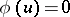(9)

one constructs an equation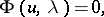(10)

depending on a parameter, such that for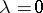a solution of (10) is known: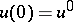, and such that for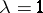the solutions of (9) and (10) are the same. For example, one can take(11)

By differentiating (1) with respect to the parameter and taking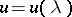one obtains a differential equation for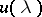; for the case (11) it takes the form(12)

By splitting the interval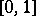intoparts by points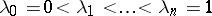and using for (12) a numerical discretization formula at the points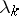(e.g., Euler's method, the Runge–Kutta method, etc.), one obtains recurrence relations between the quantities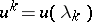, which one uses to construct the formulas of an iteration method. Thus, after e.g. applying Euler's method, (12) is replaced by the relations(13)

where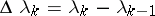, which determine the following two-step iteration method containing internal and external iteration cycles: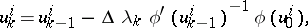(14)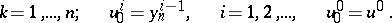For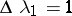andthis turns into Newton's classical method. A continuous analogue of Newton's iteration method can also be obtained in another way: In (11) the variable is replaced by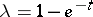. Then the differential equation (12) takes the form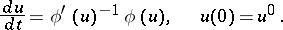(15)

Numerical integration of (15) by Euler's method with respect to the points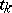leads to the iteration methodwhich coincides forwith Newton's classical method.

Continuous analogues of iteration methods for the solution of boundary value problems for the differential equations of mathematical physics are, as a rule, mixed problems for partial differential equations of a special form (e.g. with rapidly oscillating coefficients or with small coefficients in front of the highest derivatives).